• 图像插值方法比较本文介绍几种经典插值的原理，代码过程以及对性能作出比较。简单说来，在对原始图像进行缩放、旋转变换的时候，因为在目标图像中像素分布发生了变换，所以需根据一定的映射规则建立从原始图像到目标...
图像插值方法比较

本文介绍几种经典插值的原理，代码过程以及对性能作出比较。

简单说来，在对原始图像进行缩放、旋转变换的时候，因为在目标图像中像素分布发生了变换，所以需根据一定的映射规则建立从原始图像到目标图像的转换。

Nearest 差值

顾名思义，最近邻插值就是从原始目标中找到最匹配的位置。不多说，直接上代码。

static int nearestArea(Mat &src, float scale_x, float scale_y, char *argc)
{
Mat dstMat1 = Mat(src.rows * scale_y, src.cols * scale_x, src.type());
typedef BOOST_TYPEOF(*src.data) ElementType;
int WidthSrc = src.cols;
int HeightSrc = src.rows;
int WidthDst = dstMat1.cols;
int HeightDst = dstMat1.rows;
int chal_num = src.channels();
char name;
char **ful_name = &argc;
char *prefix = nullptr;
char *subfix = nullptr;
prefix = strsep(ful_name, ".");
subfix = strsep(ful_name, ".");
if (!prefix || !subfix) {
printf("Get subfix from file name failed\n");
return -1;
}
snprintf(name, sizeof(name), "%s_%.2f_%.2f_Nearest.%s", prefix, scale_x, scale_y, subfix);

clock_t start = clock();

for (int w = 0; w < WidthDst; w++) {
float rx = ((float)w + 0.5) / scale_x - 0.5;
int sx = cvFloor(rx);
sx = MIN(sx, WidthSrc - 2);
sx = MAX(0, sx);

short rx_sh_buf;
rx_sh_buf = (short)((1.f - rx) * 2048);
rx_sh_buf = 2048 - rx_sh_buf;

for (int h = 0; h < HeightDst; h++) {
float ry = ((float)h + 0.5) / scale_y - 0.5;
int sy = cvFloor(ry);
sy = MIN(sy, HeightSrc - 2);
sy = MAX(0, sy);

switch (chal_num) {
case 1:
dstMat1.at<ElementType>(h, w) =
src.at<ElementType>(sy, sx);
break;
case 2:
for (int m = 0; m < 2; m++) {
dstMat1.at<Vec<ElementType, 2>>(h, w)[m] =
src.at<Vec<ElementType, 2>>(sy, sx)[m];
}
break;
case 3:
for (int m = 0; m < 3; m++) {
dstMat1.at<Vec<ElementType, 3>>(h, w)[m] =
src.at<Vec<ElementType, 3>>(sy, sx)[m];
}
break;
default:
break;
}
}
}
clock_t end = clock();
printf("Interpolation time: %f\n", (float)(end - start) / CLOCKS_PER_SEC * 1000);
if (dstMat1.data) {
imwrite(name, dstMat1);
}

return 1;
}

Bi-linear 双线性插值

双线性插值的思路就是利用映射到原始图像匹配点像素临近四个点对目标图像的像素点进行求解。原因在于反向求解匹配像素点的时候，会产生小数，舍入之后会产生较大误差。计算思路可以用下图表示：X方向 f(R1)≈x−x1x2−x⋅f(Q21)+x2−xx2−x1⋅f(Q11)<!--//--><![CDATA[//><!--
f(R_1)\approx\frac{x-x_1}{x_2-x}\cdot f(Q_{21}) + \frac{x_2-x}{x_2-x_1}\cdot f(Q_{11})
//--><!]]>f(R2)≈x−x1x2−x⋅f(Q12+x2−xx2−x1⋅f(Q22<!--//--><![CDATA[//><!--
f(R_2)\approx\frac{x-x_1}{x_2-x}\cdot f(Q_{12} + \frac{x_2-x}{x_2-x_1}\cdot f(Q_{22}
//--><!]]>
Y方向 f(P)≈y−y1y2−y1⋅f(R1)+y2−yy2−y1⋅f(R2)<!--//--><![CDATA[//><!--
f(P)\approx\frac{y-y_1}{y_2-y_1}\cdot f(R1) + \frac{y_2 -y}{y_2-y_1}\cdot f(R_2)
//--><!]]>

将求得的像素点表示为(x+u,y+v)<!--//--><![CDATA[//><!--
(x + u, y + v)
//--><!]]>, 其中u, v表示小数部分
根据上述推导，目标像素值可以表示为： f(P)≈(1−u)(1−v)(f(x,y))+(1−u)vf(x,j+1)+u(1−v)f(x+1,y)+uvf(x,y)<!--//--><![CDATA[//><!--
f(P)\approx(1 - u)(1 - v)(f(x, y)) + (1 - u)vf(x, j + 1) + u(1 - v)f(x + 1, y) + uvf(x, y)
//--><!]]>

将上述公式转化为代码：

static int bilinear(Mat &src, float scale_x, float scale_y, char* argc)
{
Mat dstMat1 = Mat(src.rows * scale_y, src.cols * scale_x, src.type());
typedef BOOST_TYPEOF(*src.data) ElementType;//用boost 在编译阶段获得数据类型
int WidthSrc = src.cols;
int HeightSrc = src.rows;
int WidthDst = dstMat1.cols;
int HeightDst = dstMat1.rows;
int chal_num = src.channels();
char name;
char **ful_name = &argc;
char *prefix = nullptr;
char *suffix = nullptr;
prefix = strsep(ful_name, ".");
subfix = strsep(ful_name, "."); //Get Image type through suffix
if (!prefix || ! suffix) {
printf("Get suffix from file name failed\n");
return -1;
}
snprintf(name, sizeof(name), "%s_%.2f_%.2f.%s", prefix, scale_x, scale_y, suffix);

for(int w = 0; w < WidthDst; w++) {
float rx = ((float)w + 0.5) / scale_x - 0.5; //根据中心对称，向左上平移，优化插值
int sx = cvFloor(rx);
rx -= sx;
sx = MIN(sx, WidthSrc - 2);
sx = MAX(0, sx);

for (int h = 0; h < HeightDst; h++) {
float ry = ((float)h + 0.5) / scale_y - 0.5;
int sy = cvFloor(ry);
ry -= sy;
sy = MIN(sy, HeightSrc - 2);
sy = MAX(0, sy);

switch (chal_num) {
case 1:
dstMat1.at<ElementType>(h, w) =
(1 - rx)*(1 - ry)*(src.at<ElementType>(sy, sx)) +
(1 - rx)*ry*(src.at<ElementType>(sy, sx + 1)) +
rx*(1 - ry)*(src.at<ElementType>(sy + 1, sx)) +
rx*ry*(src.at<ElementType>(sy + 1, sx + 1));
break;
case 2:
for (int m = 0; m < 2; m++) {
dstMat1.at<Vec<ElementType, 2>>(h, w)[m] =
(1 - rx)*(1 - ry)*(src.at<Vec<ElementType, 2>>(sy, sx)[m]) +
(1 - rx)*ry*(src.at<Vec<ElementType, 2>>(sy, sx + 1)[m]) +
rx*(1 - ry)*(src.at<Vec<ElementType, 2>>(sy + 1, sx)[m]) +
rx*ry*(src.at<Vec<ElementType, 2>>(sy + 1, sx + 1)[m]);
}
break;
case 3:
for (int m = 0; m < 3; m++) {
dstMat1.at<Vec<ElementType, 3>>(h, w)[m] =
(1 - rx)*(1 - ry)*(src.at<Vec<ElementType, 3>>(sy, sx)[m]) +
(1 - rx)*ry*(src.at<Vec<ElementType, 3>>(sy, sx + 1)[m]) +
rx*(1 - ry)*(src.at<Vec<ElementType, 3>>(sy + 1, sx)[m]) +
rx*ry*(src.at<Vec<ElementType, 3>>(sy + 1, sx + 1)[m]);
}
}

}
}
if (dstMat1.data) {
imwrite(name, dstMat1);
}

return 1;
}

本质上插值的过程是目标图像到原始图像的映射，由此需要保证的一点就是前后两者之间的中心点应该是对齐的。实际运算中，目标图像像素值计算有可能会在原始图像中有所偏移。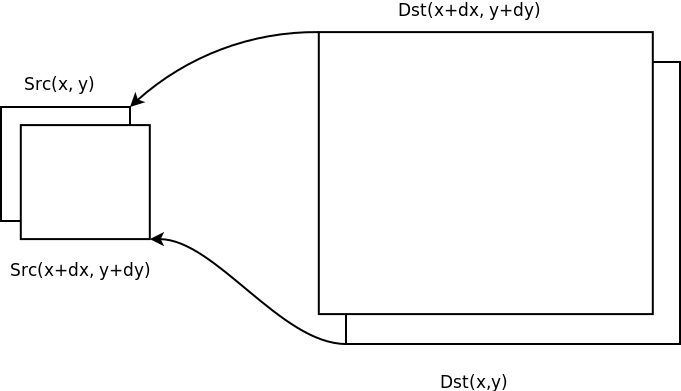查看上图，因为直接计算会导致目标图像映射到原始图像的像素值向左上角偏移，所以在计算之前做了一个(dx,dy)<!--//--><![CDATA[//><!--
(dx, dy)
//--><!]]>偏移， 实际过程中取dx=0.5,dy=0.5<!--//--><![CDATA[//><!--
dx = 0.5, dy = 0.5
//--><!]]>。
展开全文opencv 性能
• ## 常见图像插值算法的原理与C++实现

千次阅读 多人点赞 2020-12-22 23:17:47
插值之前，首先讲像素重采样的概念。假设有图像A和图像B，其中A为源图像，B为目标图像，A与B的坐标具有对应关系f：(xa, ya)=f(xb, yb)通过关系f，把A的像素值赋值给B中...


讲插值之前，首先讲像素重采样的概念。假设有图像A和图像B，其中A为源图像，B为目标图像，A与B的坐标具有对应关系f：(xa, ya)=f(xb, yb)通过关系f，把A的像素值赋值给B中对应像素点的过程，叫做图像A的像素重采样，图像B为重采样之后的图像。比如对于B的任意像素点(x, y)，其对应的A的像素点为(x', y')，那么则把A中点(x', y')的像素值A(x', y')赋值给B中点(x, y)的像素值B(x, y)。(x‘, y’)=f(x, y)B(x, y)=A(x', y')像素重采样的常见应用场景为图像缩放和图像配准。在实际应用过程中，源图像A的对应坐标往往不是整数，而是浮点型数据（如下图所示），因此不能直接取其像素值赋值给目标图像上的对应点。此时要获取点A(x', y')的像素值，插值算法就派上用场了。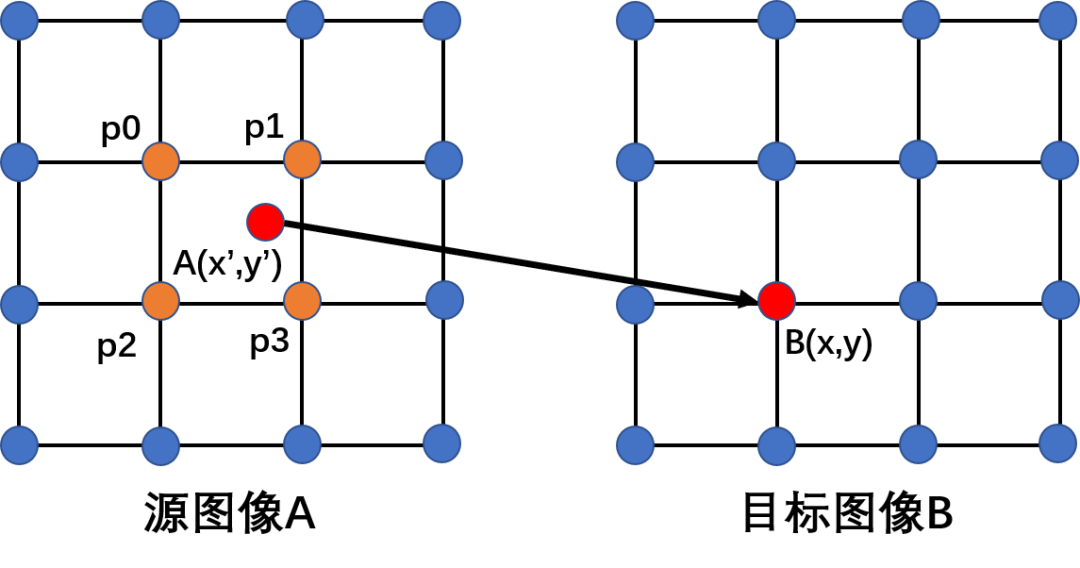所谓插值，就是使用浮点型坐标点的周围整型点的像素值来计算该浮点型坐标点的像素值。比如上图中，点(x', y')为浮点型坐标点，使用其周围整型点p0、p1、p2、p3的像素值来计算其像素值A(x', y')，这个过程就是插值。常见的插值算法有最邻近插值、双线性插值、双三次插值，不管什么插值算法，其本质都是取浮点型坐标点周围的n*n个整型坐标点的像素值进行加权和，从而得到该浮点型坐标点的像素值，如下式：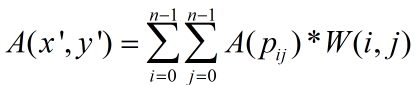不同插值算法的区别在于权重W的计算不一样，下文将分别详细讲解最邻近插值、双线性插值、双三次插值的计算原理与实现。1. 最邻近插值最邻近插值取离浮点型坐标点的最近点的像素值作为其像素值，也可以看成使用浮点型坐标点周围2*2个整型点的像素值来计算其像素值，不过只有最靠近的那个点权重为1，其余3个点权重系数都为0。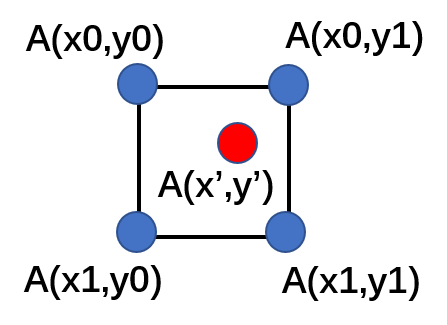插值的计算如下式：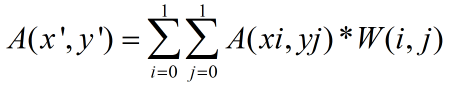其权重计算如下式：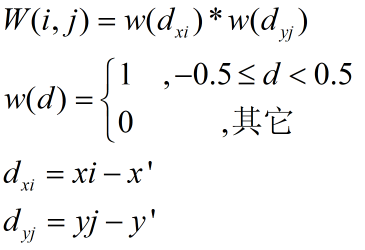最邻近插值的代码实现最简单，直接对浮点型坐标进行四舍五入取整即可,假设浮点坐标为(x_float, y_float)，那么使用最邻近插值计算A(x_float, y_float)的代码实现如下：int x = (int)(x_float + 0.5);  //加0.5再截断取整，与四舍五入取整等效
int y = (int)(y_float + 0.5);
uchar inner_value = A.ptr<uchar>(y)[x];  //A(x',y')=A(x,y)
2. 双线性插值双线性插值与最邻近插值类似，同样使用浮点型坐标点周围2*2个整型点的像素值来计算其像素值，不过其周围每个整型点的权重都不为0，也就是说，其权重计算与最邻近插值不一样：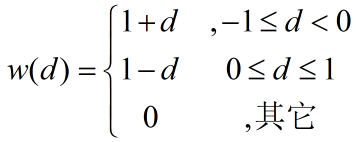浮点坐标点(x_float, y_float)双线性插值的代码实现如下：int x0 = floor(x_float);
int x1 = x0 + 1;
int y0 = floor(y_float);
int y1 = y0 + 1;

float fracRow = y_float - y0;  //求浮点坐标的小数部分
float fracCol = x_float - x0;

float k0 = 1.0 - fracRow;
float k1 = 1.0 - fracCol;
float w0 = k0*k1;
float w1 = fracRow*k1;
float w2 = k0*fracCol;
float w3 = fracRow*fracCol;

uchar inner_value = (uchar)(w0 * A.ptr<uchar>(y0)[x0] + w1 * A.ptr<uchar>(y1)[x0] + w2 * A.ptr<uchar>(y0)[x1] + w3 * A.ptr<uchar>(y1)[x1]);
3. 双三次插值双三次插值使用浮点型坐标点周围4*4个整型点的像素值来计算其像素值，如下图所示：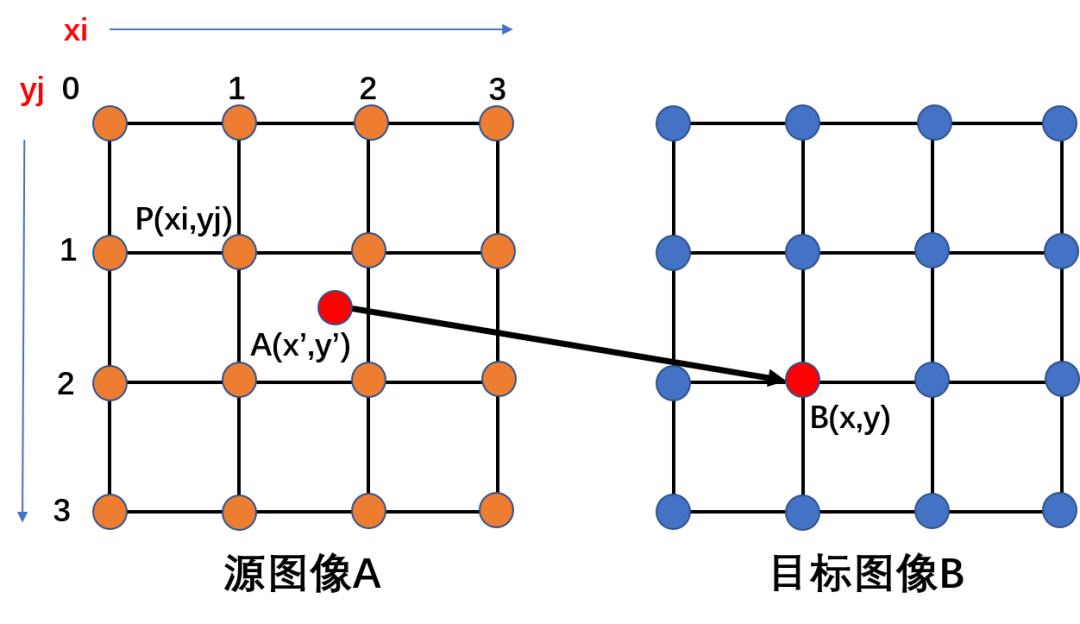浮点型坐标点的插值为其周围4*4整型坐标点像素值的加权和：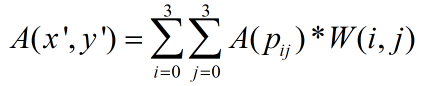其中权重W(i,j)的计算如下式，其中a取值范围-1~0之间，一般取固定值-0.5。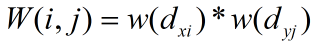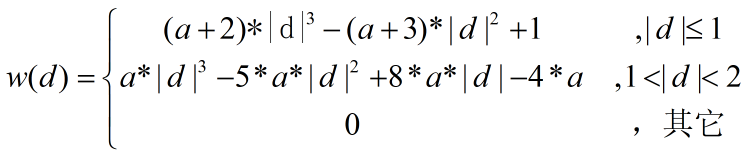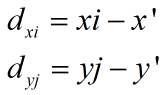双三次插值的实现代码如下。首先是权重函数的实现：float cubic_coeff(float x, float a)
{
if(x <= 1)
{
return 1-(a+3)*x*x+(a+2)*x*x*x;
}
else if(x < 2)
{
return -4*a+8*a*x-5*a*x*x+a*x*x*x;
}
return 0.0;
}
接着是权重系数的计算实现：void cal_cubic_coeff(float x, float y, float *coeff)
{
/*calc the coeff*/
float u = x - floor(x);
float v = y - floor(y);

u += 1;
v += 1;
float a = -0.15;
float a_mul_4 = (a + a) + (a + a);
float a_mul_5 = a_mul_4 + a;
float a_mul_8 = a_mul_4 + a_mul_4;
float a_add_3 = a + 3;
float a_add_2 = a + 2;

float A;
A = cubic_coeff(abs(u), a);
A = cubic_coeff(abs(u-1), a);
A = cubic_coeff(abs(u-2), a);
A = cubic_coeff(abs(u-3), a);

for (int s = 0; s < 4; s++)
{
float C = cubic_coeff(abs(v-s), a);
coeff[s*4] = A*C;
coeff[s*4+1] = A*C;
coeff[s*4+2] = A*C;
coeff[s*4+3] = A*C;
}
}
最后，是双三次插值代码：uchar cubic_inner(Mat A, float x_float, float y_float, float a)
{
float coeff;
cal_cubic_coeff(x_float, y_float, coeff);  //计算权重系数

float sum = 0.0;
int x0 = floor(x_float) - 1;
int y0 = floor(y_float) - 1;

for(int i = 0; i < 4; i++)
{
for(int j = 0; j < 4; j++)
{
sum += coeff[i*4+j]*A.ptr<uchar>(y0+i)[x0+j];
}
}

uchar inner_value = (uchar)sum;
return inner_value;
}
从插值效果来说：双三次插值>双线性插值>最邻近插值，从计算复杂度来说，同样是：双三次插值>双线性插值>最邻近插值。所以实际使用时，根据自己的需要选择合适的插值算法。欢迎扫码关注以下微信公众号，接下来会不定时更新更加精彩的内容噢～展开全文算法 计算机视觉 opencv python java
• OpenCV图像缩放resize各种插值方式的比较 目录 OpenCV图像缩放resize各种插值方式的比较 1. resize函数说明 2.各种插值方式的比较 2.1 INTER_NEAREST（最近邻插值） 2.2 INTER_CUBIC (三次样条插值) 2.3 ...
OpenCV图像缩放resize各种插值方式的比较

目录

OpenCV图像缩放resize各种插值方式的比较

1. resize函数说明

2.各种插值方式的比较

2.1 INTER_NEAREST（最近邻插值）

2.2 INTER_CUBIC  (三次样条插值)

2.3 INTER_LINEAR(线性插值)

2.4 INTER_AREA  (区域插值)

3. 总结

1. resize函数说明

OpenCV提供了resize函数来改变图像的大小，函数原型如下：

void resize(InputArray src, OutputArray dst, Size dsize, double fx=0, double fy=0, int interpolation=INTER_LINEAR );


参数说明：

src：输入，原图像，即待改变大小的图像；
dst：输出，改变大小之后的图像，这个图像和原图像具有相同的内容，只是大小和原图像不一样而已；
dsize：输出图像的大小。如果这个参数不为0，那么就代表将原图像缩放到这个Size(width，height)指定的大小；如果这个参数为0，那么原图像缩放之后的大小就要通过下面的公式来计算：
dsize = Size(round(fx*src.cols), round(fy*src.rows))

其中，fx和fy就是下面要说的两个参数，是图像width方向和height方向的缩放比例。

fx：width方向的缩放比例，如果它是0，那么它就会按照(double)dsize.width/src.cols来计算；
fy：height方向的缩放比例，如果它是0，那么它就会按照(double)dsize.height/src.rows来计算；
interpolation：这个是指定插值的方式，图像缩放之后，肯定像素要进行重新计算的，就靠这个参数来指定重新计算像素的方式，有以下几种：
INTER_NEAREST - 最邻近插值
INTER_LINEAR - 双线性插值，如果最后一个参数你不指定，默认使用这种方法
INTER_AREA -区域插值 resampling using pixel area relation. It may be a preferred method for image decimation, as it gives moire’-free results. But when the image is zoomed, it is similar to the INTER_NEAREST method.
INTER_CUBIC - 4x4像素邻域内的双立方插值
INTER_LANCZOS4 - 8x8像素邻域内的Lanczos插值

使用注意事项：

dsize和fx/fy不能同时为0，要么你就指定好dsize的值，让fx和fy空置直接使用默认值，就像

resize(img, imgDst, Size(30,30));

要么你就让dsize为0，指定好fx和fy的值，比如fx=fy=0.5，那么就相当于把原图两个方向缩小一倍！

OpenCV官方说明：注意红色方框那句话：https://docs.opencv.org/3.2.0/da/d54/group__imgproc__transform.html#ga47a974309e9102f5f08231edc7e7529d

To shrink an image, it will generally look best with cv::INTER_AREA interpolation, whereas to enlarge an image, it will generally look best with cv::INTER_CUBIC (slow) or cv::INTER_LINEAR (faster but still looks OK).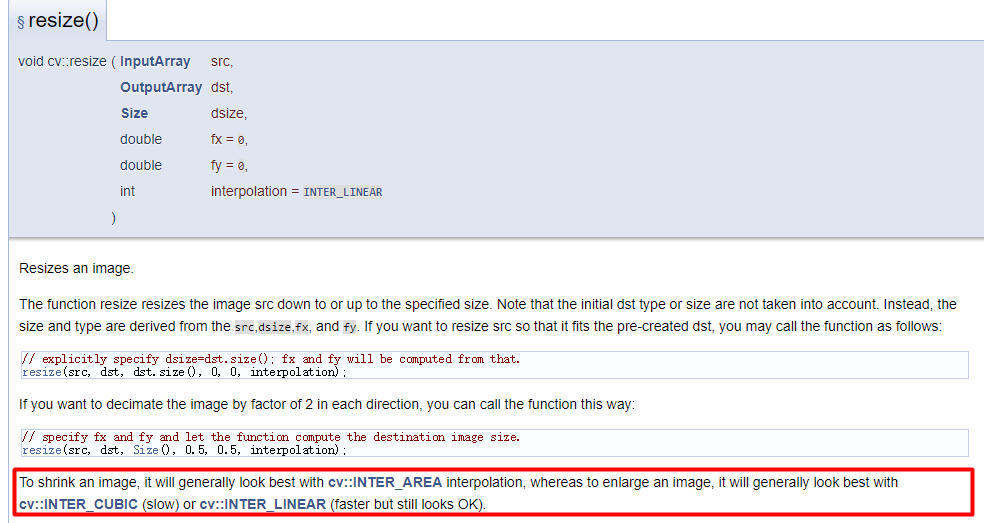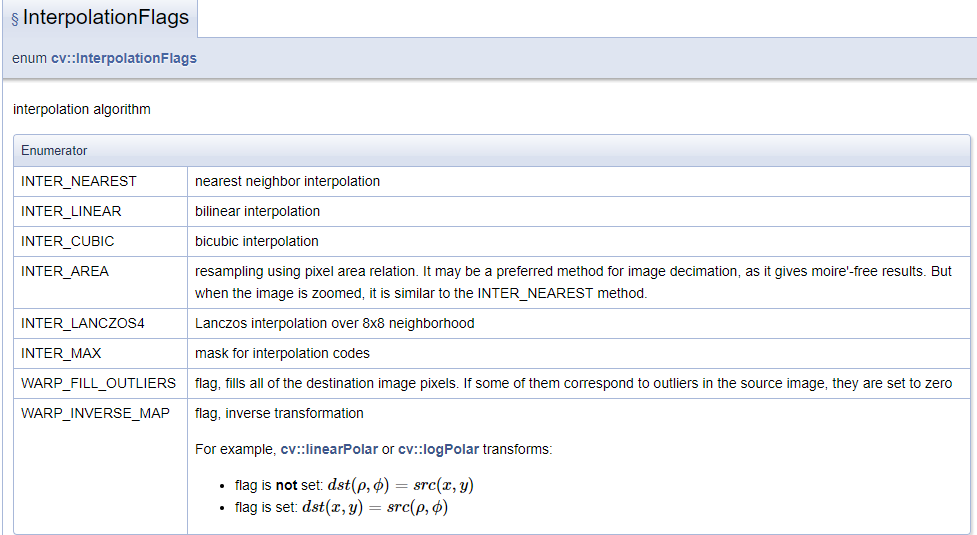2.各种插值方式的比较

OpenCV的cv::resize函数支持多种插值方式，这里主要比较下面四个常用的插值方式。

参考资料：《OpenCV中resize函数五种插值算法的实现过程》https://blog.csdn.net/fengbingchun/article/details/17335477

2.1 INTER_NEAREST（最近邻插值）

最近邻插值是最简单的插值方法，选取离目标点最近的点作为新的插入点，计算公式表示如下：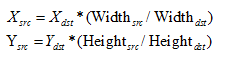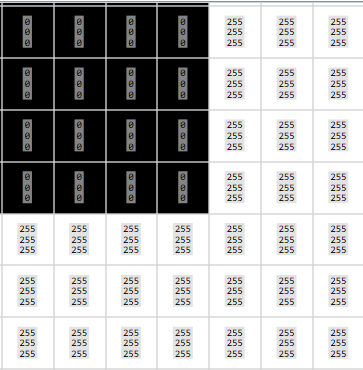插值后的边缘效果：由于是以最近的点作为新的插入点，因此边缘不会出现缓慢的渐慢过度区域，这也导致放大的图像容易出现锯齿的现象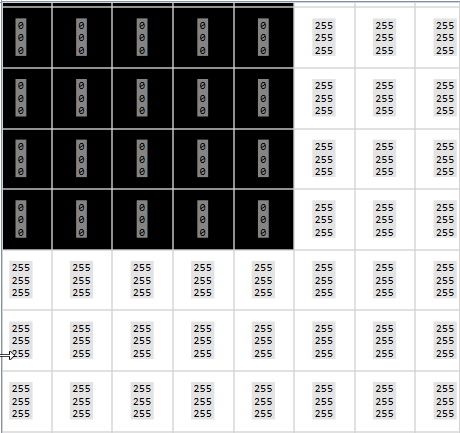2.2 INTER_CUBIC  (三次样条插值)插值后的边缘效果：可以有效避免出现锯齿的现象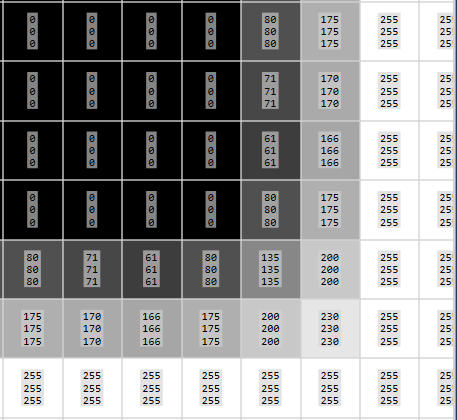2.3 INTER_LINEAR(线性插值)

线性插值是以距离为权重的一种插值方式。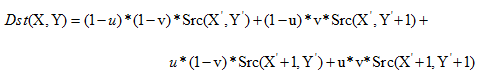插值后的边缘效果：可以有效避免出现锯齿的现象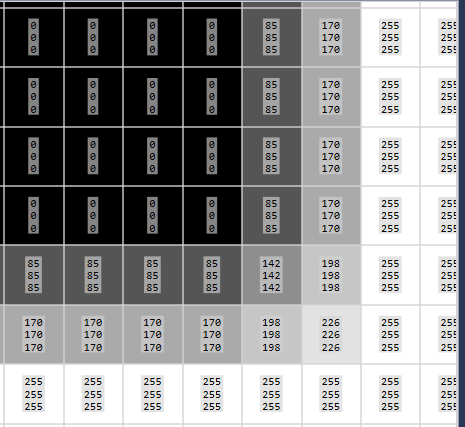2.4 INTER_AREA  (区域插值)

区域插值共分三种情况，图像放大时类似于双线性插值，图像缩小(x轴、y轴同时缩小)又分两种情况，此情况下可以避免波纹出现。因此对图像进行缩小时，为了避免出现波纹现象，推荐采用区域插值方法。

OpenGL说明文档有这么解释：To shrink an image, it will generally look best with #INTER_AREA interpolation, whereas to enlarge an image, it will generally look best with #INTER_CUBIC (slow) or #INTER_LINEAR (faster but still looks OK).

如果要缩小图像，通常推荐使用INTER_AREA插值效果最好，而要放大图像，通常使用INTER_CUBIC(速度较慢，但效果最好)，或者使用INTER_LINEAR(速度较快，效果还可以)。插值后的边缘效果：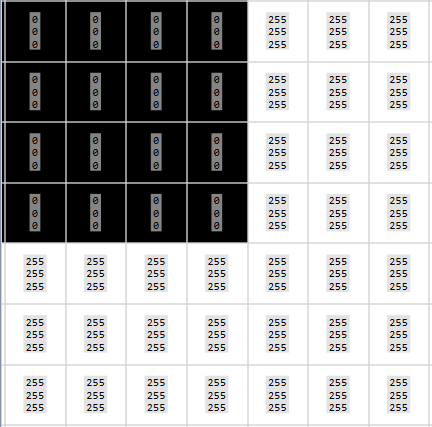测试代码：

#include <chrono>
#include <opencv2/opencv.hpp>
#define  millisecond 1000000
#define DEBUG_PRINT(...)  printf( __VA_ARGS__); printf("\n")
#define DEBUG_TIME(time_) auto time_ =std::chrono::high_resolution_clock::now()
#define RUN_TIME(time_)  (double)(time_).count()/millisecond
using namespace std;

cv::Mat image_resize(cv::Mat image, int width, int height, int interpolation, int num) {
cv::Mat dest;
for (int i = 0; i < num; ++i) {
cv::resize(image, dest, cv::Size(width, height), 0, 0, interpolation);//最近邻插值
}
return dest;
}

int main() {
string path = "../1.jpg";
cv::resize(image, image, cv::Size(1000, 1000));
int re_width = 900;
int re_height = 900;
int  num=10;
cv::Mat image2X_INTER_NEAREST;
cv::Mat image2X_INTER_LINEAR;
cv::Mat image2X_INTER_AREA;
cv::Mat image2X_INTER_CUBIC;
cv::Mat initMat;
DEBUG_PRINT("image input size:%dx%d", image.rows, image.cols);
DEBUG_TIME(T0);
image2X_INTER_NEAREST=image_resize(image, re_width, re_height, cv::INTER_NEAREST, num);
DEBUG_TIME(T1);
image2X_INTER_LINEAR=image_resize(image, re_width, re_height, cv::INTER_LINEAR, num);
DEBUG_TIME(T2);
image2X_INTER_AREA=image_resize(image, re_width, re_height, cv::INTER_AREA, num);
DEBUG_TIME(T3);
image2X_INTER_CUBIC=image_resize(image, re_width, re_height, cv::INTER_CUBIC, num);
DEBUG_TIME(T4);
DEBUG_PRINT("resize_image:%dx%d,INTER_NEAREST:%3.3fms",
image2X_INTER_NEAREST.rows,
image2X_INTER_NEAREST.cols,
RUN_TIME(T1 - T0)/num);
DEBUG_PRINT("resize_image:%dx%d,INTER_LINEAR :%3.3fms",
image2X_INTER_LINEAR.rows,
image2X_INTER_LINEAR.cols,
RUN_TIME(T2 - T1)/num);
DEBUG_PRINT("resize_image:%dx%d,INTER_AREA   :%3.3fms",
image2X_INTER_AREA.rows,
image2X_INTER_AREA.cols,
RUN_TIME(T3 - T2)/num);
DEBUG_PRINT("resize_image:%dx%d,INTER_CUBIC  :%3.3fms",
image2X_INTER_CUBIC.rows,
image2X_INTER_CUBIC.cols,
RUN_TIME(T4 - T3)/num);
return 0;
}



运行结果：

image input size:1000x1000
resize_image:900x900,INTER_NEAREST:0.389ms
resize_image:900x900,INTER_LINEAR :0.605ms
resize_image:900x900,INTER_AREA   :2.611ms
resize_image:900x900,INTER_CUBIC  :1.920ms

3. 总结

测试结果表明：

速度比较：INTER_NEAREST（最近邻插值)>INTER_LINEAR(线性插值)>INTER_CUBIC(三次样条插值)>INTER_AREA  (区域插值)
对图像进行缩小时，为了避免出现波纹现象，推荐采用INTER_AREA 区域插值方法。
OpenCV推荐：如果要缩小图像，通常推荐使用#INTER_AREA插值效果最好，而要放大图像，通常使用INTER_CUBIC(速度较慢，但效果最好)，或者使用INTER_LINEAR(速度较快，效果还可以)。至于最近邻插值INTER_NEAREST，一般不推荐使用


展开全文• ## 图像插值

千次阅读 2016-03-30 21:14:44
数字图像插值 数字图像插值——bayer去马赛克或者是图像放大。将图像中的一个像素点改变或者映射到另外一个位置。图像大小变换：需要增加或者减少总像素点数；映射：镜头畸变校正，改变的角度，和旋转图像。 ...
数字图像插值

数字图像插值——bayer去马赛克或者是图像放大。将图像中的一个像素点改变或者映射到另外一个位置。图像大小变换：需要增加或者减少总像素点数；映射：镜头畸变校正，改变的角度，和旋转图像。内容：

插值是使用已知的数据估计未知的点。如下所示。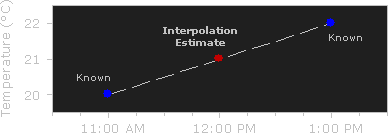使用二次插值:图像缩放的例子

图像插值在两个方向进行, 根据周围像素点实现最佳的估计。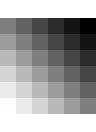Original

Enlarge 183%→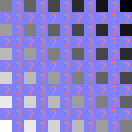Before Interpolation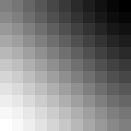After Interpolation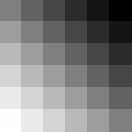No Interpolation

图像旋转的例子
图像细节严重损失：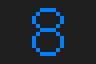Original

Rotation→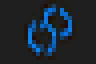45° Rotation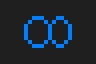90° Rotation
(Lossless)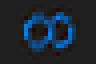2 X 45°
Rotations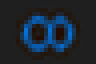6 X 15°
Rotations

90° 方向没损失，是因为没有边缘像素被分为两个。一般不要超过一次旋转。
上面的结果叫 "bicubic" algorithm（双三次算法）。
插值算法的类型：
一般分为两类: 自适应和非自适应。自适应的方法可以根据插值的内容来改变（尖锐的边缘或者是平滑的纹理），非自适应的方法对所有的像素点都进行同样的处理。
非自适应算法包括: 最邻近方法, 双线性, 双三次, 样条, sinc, lanczos 和其他。由于其复杂度, 这些插值的时候使用从0 to 256 (or more) 邻近像素。 包含越多的邻近像素，他们越精确，但是花费的时间也越长。这些算法可以用来扭曲和缩放照片。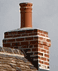Original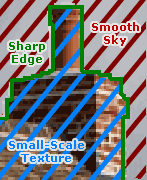Enlarged 250%

自适应算法包含许多专利，如: Qimage, PhotoZoom Pro, Genuine Fractals和其他。许多应用他们插值的不同版本 (on a pixel-by-pixel basis)当他们检测边缘时 —目标是最小化插值干扰。

最邻近插值

最邻近算法在所有插值算法中时间最短，因为它只考虑一个像素点—离待插像素点最近的像素点。
双线性插值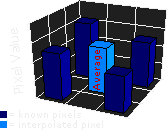双线性插值考虑待插像素最近的 2x2 已知像素点。需要加权四个像素值来求得最终的像素值。这使得插值出来比最邻近插值平滑。

双三次插值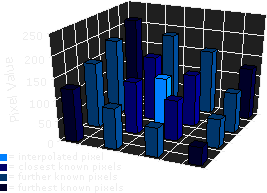基于双线性插值，考虑最近的 4x4已知像素点 —总共16个像素点。由于离待插像素点的距离不同， 在计算中距离近的像素给出的权重较大。双三次产生的图像比前两次的尖锐，有理想的处理时间和输出质量。因此，在很多图像编辑程序中是标准算法 (包括 Adobe Photoshop), 打印机和相机插值。
高阶插值: SPLINE & SINC
有许多其他的插值算法需要考虑更多的相邻像素点，因此复杂度更高。 这些算法包括 spline and sinc,在插值后可以恢复大多数图像信息。当图像要求几倍变换时，它们很有用。然而，对于单步放大或旋转，这些高阶算法提供递减的视觉提升，并且处理时间增加。
注意插值干扰
所有的非自适应插值试图在三个干扰中寻求最佳平衡： edge halos（边缘光晕）, blurring（模糊） and aliasing（混叠）.Original

Enlarged
400%→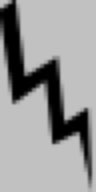AliasingBlurringEdge Halo

所有先进的非自适应插值以牺牲两种干扰为条件提升一种干扰的性能。
自适应插值可能不会产生上面的干扰，它们可能包含非图像纹理或者是奇怪的像素点（小范围）。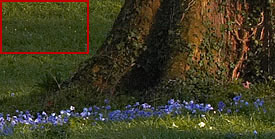Original

Enlarged
220%→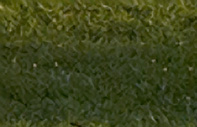Adaptive Interpolation

另一方面，来自自适应插值的一些 "干扰"被视为是有益的。

抗锯齿
抗锯齿是最小化锯齿状斜边缘的出现。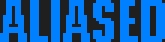Enlarged 300%
(With Aliasing)Enlarged 300%
(Without Aliasing)

抗锯齿移除了这些锯齿，并且平滑了边缘，提高了分辨率。它考虑理想边缘与相邻像素重合多少。锯齿边缘简单的提升或者降低非中间值，抗锯齿边缘, 给出一个值来优化在每个像素内包含多少边缘。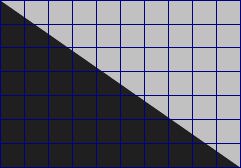Perfect Diagonal

Choose:
Aliased
Anti-Aliased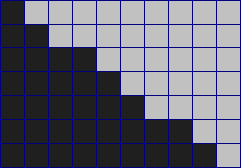Resampled to Low Resolution

A major obstacle when enlarging an image is preventing the interpolator from inducing or exacerbating aliasing. Many adaptive interpolators detect the presence of edges and adjust to minimize aliasing while still retaining edge
sharpness. Since an anti-aliased edge contains information about that edge's location at higher resolutions, it is also conceivable that a powerful adaptive (edge-detecting) interpolator could at least partially reconstruct this edge when enlarging.

注意光学 vs. 数码变焦
现在很多相机可以进行光学和数码变焦。一个相机通过移动zoom lens 增加光量来进行光学变焦。相反的，数码变焦通过简单的插值图像 — 传感器获得图像之后。10X Optical Zoom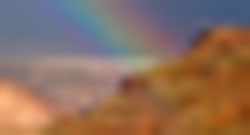10X Digital Zoom

尽管数码变焦后包含相同的像素点，细节远远比光学变焦小。应该完全避免数码变焦，除非它有助于你相机的LCD preview screen可视化一个遥远的物体。


展开全文• ## 图像插值方法

千次阅读 2015-08-20 16:18:06
一、图像插值 所谓图像插值就是一个图像数据的再生过程，利用己知采样点的灰度值计未知采样点的灰度值，在给定的空间范围内，从有限的离散采样数据复原出原来连续的图像信号。1.1怎么理解图像插值 比如，图像...
• 文章目录常见插值算法一、最邻插值算法二、线性插值三、双线性插值四、双三次(bicubic)插值 常见插值算法 在现实生活中，我们经常会遇到把图像进行放大、几何空间变换的情况等等，这些操作都需要在源图像和目标图像...
• ## 图像插值算法及其实现

万次阅读 多人点赞 2018-08-27 19:27:30
由于sensor、codec、display device都是...图像缩放算法往往基于插值实现，常见图像插值算法包括最近邻插值（Nearest-neighbor）、双线性插值（Bilinear）、双立方插值（bicubic）、lanczos插值、方向插值（Edge-d...resize scaler bilinear 缩放
• 您想使用最近邻插值在正水平方向上将此图像平移1.7像素。 以下步骤说明了块的最近邻插值算法 从而得到我们最后的结果： INTER_LINEAR 对于双线性插值，该块对每个输出像素值使用两个转换像素值的加权平均值。 ...计算机视觉 opencv
• ## 图像插值技术

千次阅读 2016-04-12 22:18:55
图像旋转、剪切、非线性变换等都需要图像插值。介绍最常用的三种数字图像插值方法（后向映射法）。 1、最邻近插值技术 　一种最简单的插值方法，不需要计算，在待求象素的四邻象素中，将距离待求象素最近的邻象素...
• 图像插值也叫图像重采样，在很多需要进行图像定位的计算中大量用到，OpenCV将图像插值的函数封装到了其与几何变换相关的函数中，并未直接暴露独立进行图像插值计算的接口，而在需要情况下我们需要对图像进行直接的...opencv 图像几何变换 双线性插值 双三次插值
• ## 图像插值运算总结

万次阅读 2015-03-24 20:33:34
**图像插值就是利用已知邻近像素点的灰度值（或RGB图像中的三色值）来产生未知像素点的灰度值，以便由原始图像再生出具有更高分辨率的图像。 图像插值常常用在图像的放缩，旋转等变换中。常用的插值运算有三种：最...
• 常见插值算法有最近邻插值算法，双线性插值算法，三次卷积等最近邻插值算法这是最简单的一种插值算法，根据目标图像插值后的图像大小）与源图像大小的比值来寻找目标图像的像素位置对应于源图像像素的位置。...最近邻插值算法 双线性插值法
• 图像插值知识点python代码c++代码 知识点 图像插值(Image Interpolation) 最常见四种插值算法 INTER_NEAREST = 0 INTER_LINEAR = 1 INTER_CUBIC = 2 INTER_LANCZOS4 = 4 相关的应用场景 几何变换、透视变换、插值...
• 图像插值： 是基于模型框架下，从低分辨率图像生成高分辨率图像的过程，用以恢复图像中所丢失信息。 图像插值的分类 插值，分为图像内插值和图像间插值。其主要应用是对图像进行放大以及旋转等操作。 图像内插值：...
•图像算法 代码实现
• ## 图像插值算法

千次阅读 2014-02-28 15:03:04
插值的定义： 设函数y=f(x)在区间[a,b]上有定义，且已知在点a≤x0 ...有时，在图像的几何变换中，比如缩放和旋转，输出图像上的像素点坐标有可能对应于原图像上几个像素点之间的位置，这个时候就算法
• 在现实生活中，我们经常会遇到把图像进行放大、几何空间变换的情况等等，这些操作都需要在源图像和目标图像之间建立一个映射规则，使得两图像像素坐标之间建立起一种对应关系，从而为目标图像的每一个像素赋值。...
• ## 图像插值算法总结

千次阅读 2019-04-18 21:06:05
在对图像resize缩放的时候常常要用到图像插值算法。 本文主要讲述最常见的三种插值算法，最近邻插值，双线性插值（默认设置），双三次插值。 1、最近邻插值算法 在四邻像素中，将距离待求像素最近的邻像素灰度赋给待...
• 简介： 在图像的处理过程中，经常需要对...最近邻插值是最简单的一种插值方式，就是在对图像进行坐标变换之后，选取原图像中距离最近的像素点进行插值（简单说就是将目标图像的像素位置映射到源图像之后，对x、y...C
• 五种插值算法，目的是用于图像扩大时超分辨率，缩小不失真，常用于放大图片 一、最近邻插值 这种方法失真严重但运算简单，缩小图片可考虑 cv2.resize(img, (0, 0), fx=倍数, fy=倍数, interpolation=cv2.INTER_...
• 数字图像处理学习（1）——图像插值Python代码实现1. 图像插值 （Image Interpolation）2. 最近邻插值法2.1 最近邻插值法2.2 最近邻插值法（Python 代码实现——图像缩小）2.3 运行结果示例3. 双线性插值法3.1 双线...1024程序员节 python
• 计算机视觉任务中，经常要使用图像插值方法来改变图像的尺寸，如图像金字塔、图像超分辨的预处理等，可以说图像插值方法是计算机视觉任务的基本操作。本文对matlab里的图像插值方法进行分析比较。 首先简单介绍...
• 计算机视觉任务中，经常要使用图像插值方法来改变图像的尺寸，如图像金字塔、图像超分辨的预处理等，可以说图像插值方法是计算机视觉任务的基本操作。本文对opencv里的图像插值方法进行分析比较。 opencv里改变图像...
• 本文将未做插值的原始图像称作源图像，源图像插值缩放K倍后的图像称作目标图像。 以下标识符的意义： 算法 双线性插值的主要思想是目标点归一化后在四周取4个最近邻点，并根据权重对其做线性函数计算，从而得到...matlab
• 本文将未做插值的原始图像称作源图像，源图像插值缩放K倍后的图像称作目标图像。 以下标识符的意义： 算法 如下图，双三次插值就是通过对周边16个点（A,B,C,…N,O,P）进行加权计算得到目标像素点的值， (dstX/K，...matlab
• 图像插值算法原理介绍1.1最近邻插值算法1.2线性插值法1.3双线性插值2.映射与插值2.1向前映射2.2向后映射2.3总结3.python插值函数 1.图像插值算法原理介绍 所谓插值算法，意思是在已有图片的情况下，在像素与像素之间...计算机视觉...